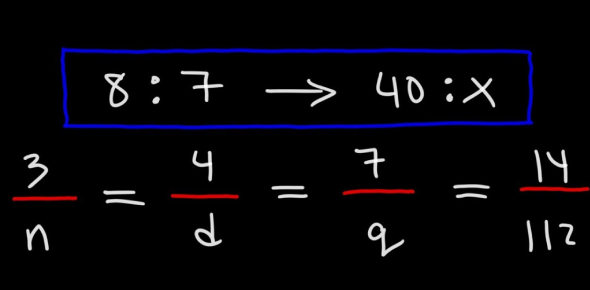# Ratio And Proportion Quiz With Answers

12 Questions | Attempts: 24614SettingsThink you're good at maths? If yes, then you must check out this amazing ratio and proportions quiz. We've designed this amazing quiz to test your mathematics skills. Students might often find it difficult to understand the topic of Ratio and proportions, but if understood well, it is the easiest and interesting topic of the mathematics subject. How well have you understood this chapter? Let's find out. Here, we will ask you a few multiple choices based questions and you've to pick the correct answer for each question. Your final score will be displayed at the end. So, are you ready to start the test? All the best!

• 1.
Matt sold 35 tickets to the school play and Renee sold 45 tickets. What is the ratio of the number of tickets Matt sold to the number of tickets Renee sold?
• A.

9/7

• B.

7/16

• C.

7/9

• D.

16/7

• 2.
Which ratios are equal to 4 : 32?
• A.

2 : 96; 2 : 16

• B.

12 : 96; 2 : 16

• C.

12 : 96; 12 : 16

• D.

2 : 96; 12 : 16

• 3.
Find a ratio equivalent to .
• A.

2/4

• B.

5/9

• C.

5/8

• D.

9/5

• 4.
Find a ratio equivalent to .
• A.

9/21

• B.

24/9

• C.

15/40

• D.

15/45

• 5.
Write the ratio of 96 runners to 216 swimmers in simplest form.
• A.

96/216

• B.

16/36

• C.

4/9

• D.

9/4

• 6.
Write the ratio of 2 cups to 3 qt as a fraction in simplest form.
• A.

3/2

• B.

2/3

• C.

2/12

• D.

1/6

• 7.
Find a ratio equivalent to 3:25.
• A.

1:9

• B.

9:225

• C.

9:75

• D.

75:9

• 8.
Which fraction shows the ratio of 3 dogs to 5 dogs?
• A.

6/10

• B.

9/10

• C.

27:40

• D.

12:15

• 9.
Find a ratio equivalent to 27/81.
• A.

3:10

• B.

9:9

• C.

1:3

• D.

5:9

• 10.
12/24 = 50/100
• A.

True

• B.

False

• 11.
1/3 = 2/9
• A.

True

• B.

False

• 12.
2/3 = 24/36
• A.

True

• B.

False

## Related TopicsBack to top
×

Wait!
Here's an interesting quiz for you.